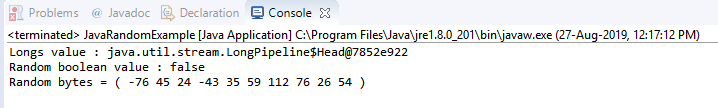# How to Generate Random Numbers using Random Class in Java?

Published on Aug 28,2019 180 Views43 / 72 Blog from Java Core Concepts

The goal of a Java Random Class is to produce a stream of pseudo-random numbers. Random Class in Java generates random numbers of different data types such as int, float, long, boolean and double. Let us dig a bit deeper and understand the concept in detail.

Below mentioned pointers will be our topics of discussion for this article:

We shall begin!

## What is a Random class in Java?

In Java, Random class is a part of java.util package. The generation of random numbers takes place by using an instance of the Java Random Class. This class provides different methods in order to produce random numbers of type integer, double, long, float, etc.

## Constructors used in a Java Random class

This class contains two constructors that are mentioned below:

• Random(): this constructor helps in creating a new random generator
• Random(long seed): this constructor helps in creating a new random generator using specified seed

Note: Whenever a random number generation process takes place, the seed value is considered. If the seed value is not provided, it gets created from system nano time. If in case 2 random instances possess the same seed value, the same sequence of random numbers will be generated.

Now, let’s check out how a method is used in a Random Class.

## Methods used in a Java random class

Some important methods are:

 Method Functionality nextDouble() Returns the next pseudo-random number that is a double value between the range of 0.0 to 1.0. nextBoolean() Returns the next pseudo-random which is a Boolean value from random number generator sequence nextFloat() Returns the next pseudo-random which is a float value between 0.0 to 1.0 nextInt() Returns the next pseudo-random which is an integer value from random number generator sequence nextInt(Int n) Returns the next pseudo-random which is an integer value between 0 and the specified value from random number generator sequence nextBytes(byte[] bytes) Generates random bytes and places them into a byte array supplied by the user Longs() Returns an unlimited stream of pseudorandom long values nextGaussian() Helps in returning the next pseudo-random, Gaussian (precisely) distributed double value with mean 0.0 and standard deviation 1.0 from this random number generator’s sequence

There are other methods that are inherited from java.lang.object like: notify, notifyAll, wait, toString, finalise, equals, clone, getClass, and hashCode.

Let’s move forward and see how a java random class is implemented in a Java program.

## Java program to represent the usage of random class

```package MyPackage;
import java.util.Random;

public class JavaRandomExample {
public static void main(String[] args) {
//create random object
Random random= new Random();
//returns unlimited stream of pseudorandom long values
System.out.println("Longs value : "+random.longs());
// Returns the next pseudorandom boolean value
boolean val = random.nextBoolean();
System.out.println("Random boolean value : "+val);
byte[] bytes = new byte;
//generates random bytes and put them in an array
random.nextBytes(bytes);
System.out.print("Random bytes = ( ");
for(int i = 0;i<bytes.length; i++)
{
System.out.printf("%d ", bytes[i]);
}
System.out.print(")");
}

}
```

Output:This brings us to the end of this article on Random Class in Java. I hope the content explained above added value to your Java knowledge. We will keep exploring the Java world. Stay tuned!

Make sure you practice as much as possible and revert your experience.

Check out the Java Training by Edureka, a trusted online learning company with a network of more than 250,000 satisfied learners spread across the globe. We are here to help you with every step on your journey, for becoming a besides this java interview questions, we come up with a curriculum which is designed for students and professionals who want to be a Java Developer.

Got a question for us? Please mention it in the comments section of this article and we will get back to you as soon as possible.REGISTER FOR FREE WEBINARThank you for registering Join Edureka Meetup community for 100+ Free Webinars each month